CBSE Class 10 Sample Paper for 2024 Boards - Maths Standard

Class 10
Solutions of Sample Papers for Class 10 Boards

## The median of the following data is 50. Find the values of ‘p’ and ‘q’, if the sum of all frequencies is 90. Also find the mode of the data.

 Marks obtained Number of students 20 - 30 p 30 - 40 15 40 - 50 25 50 - 60 20 60 - 70 q 70 - 80 8 80 - 90 10

This question is similar to Ex 13.3, 2 – Chapter 13 Class 10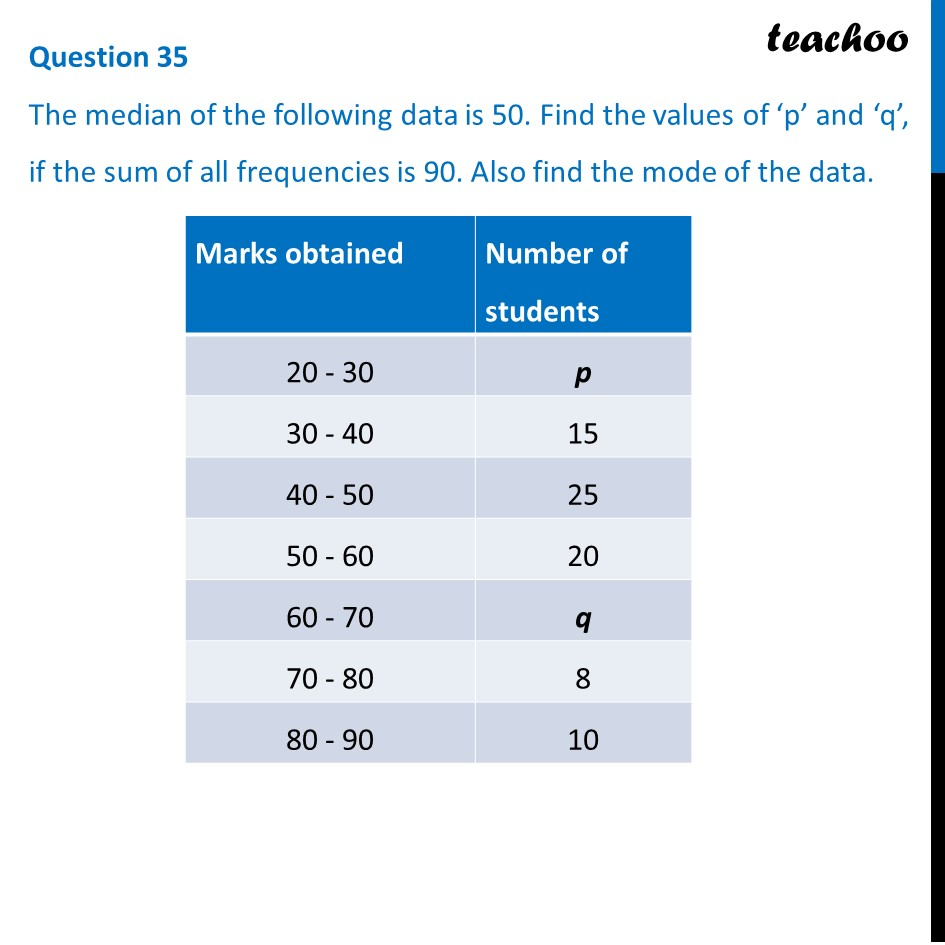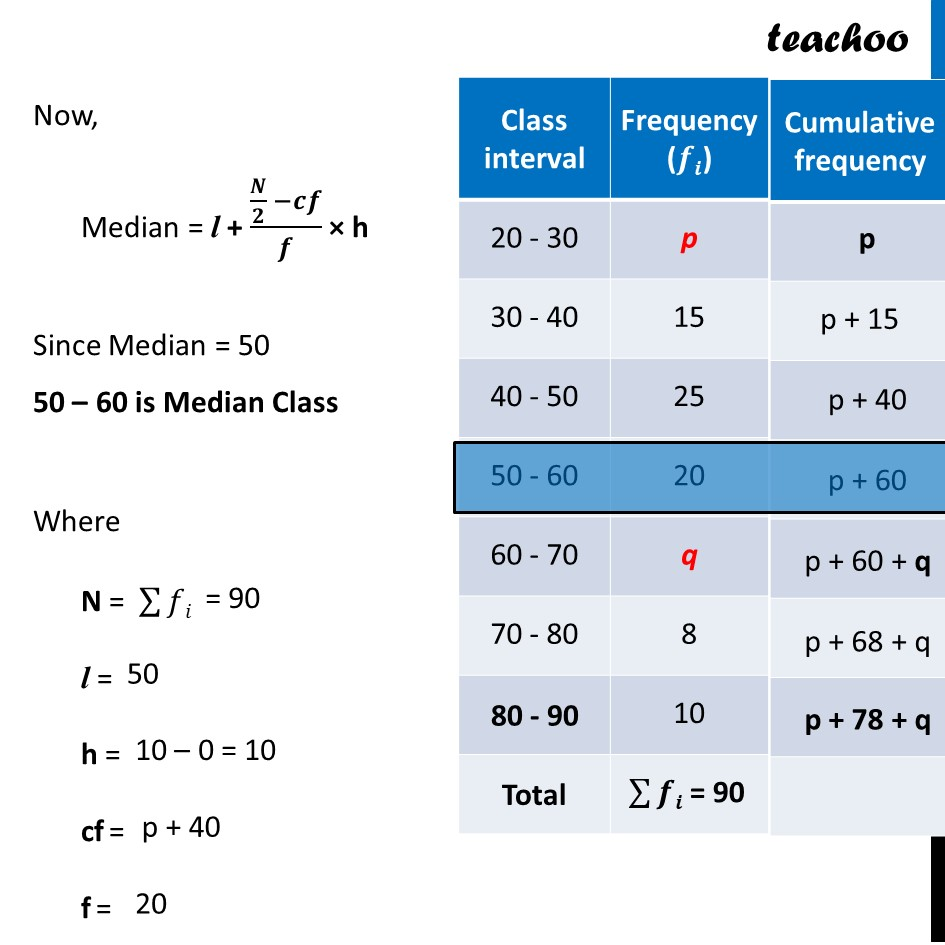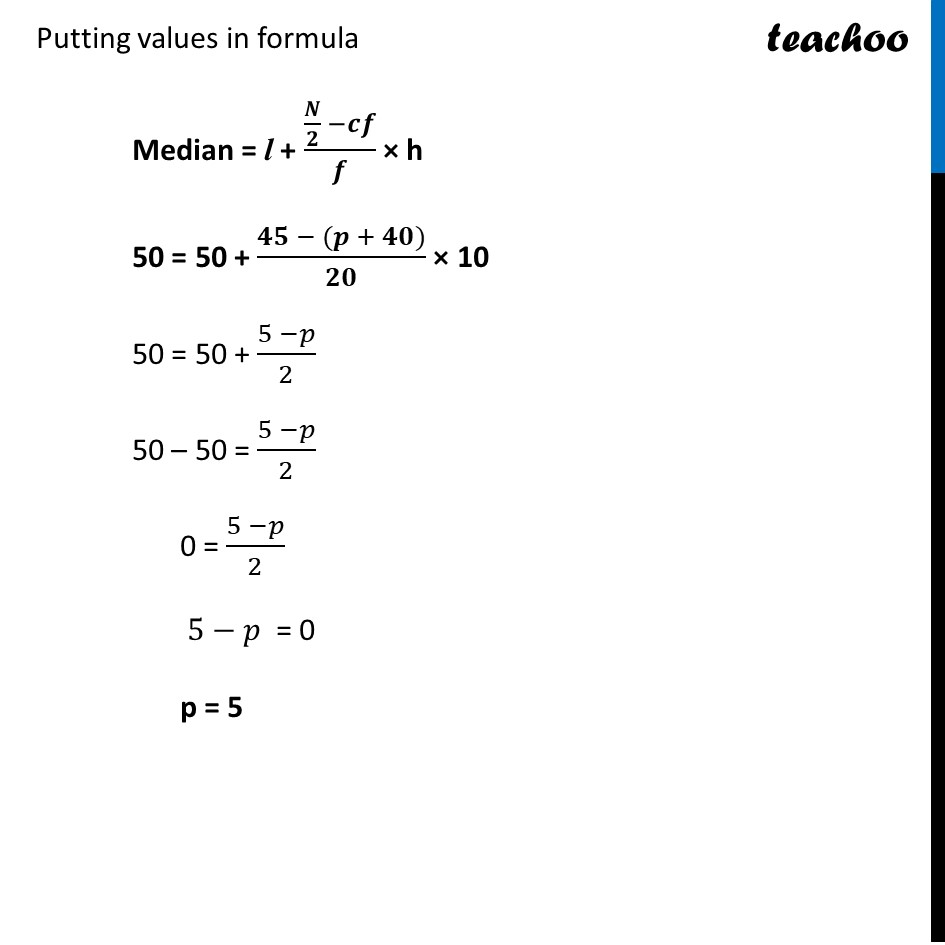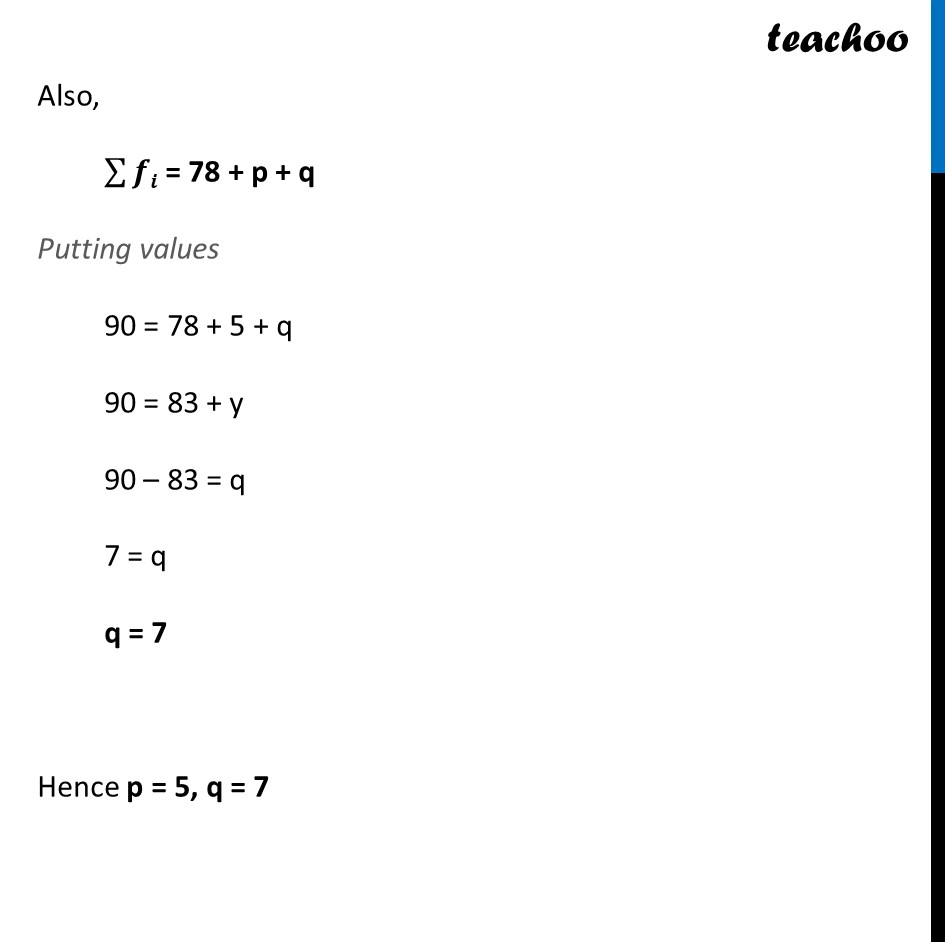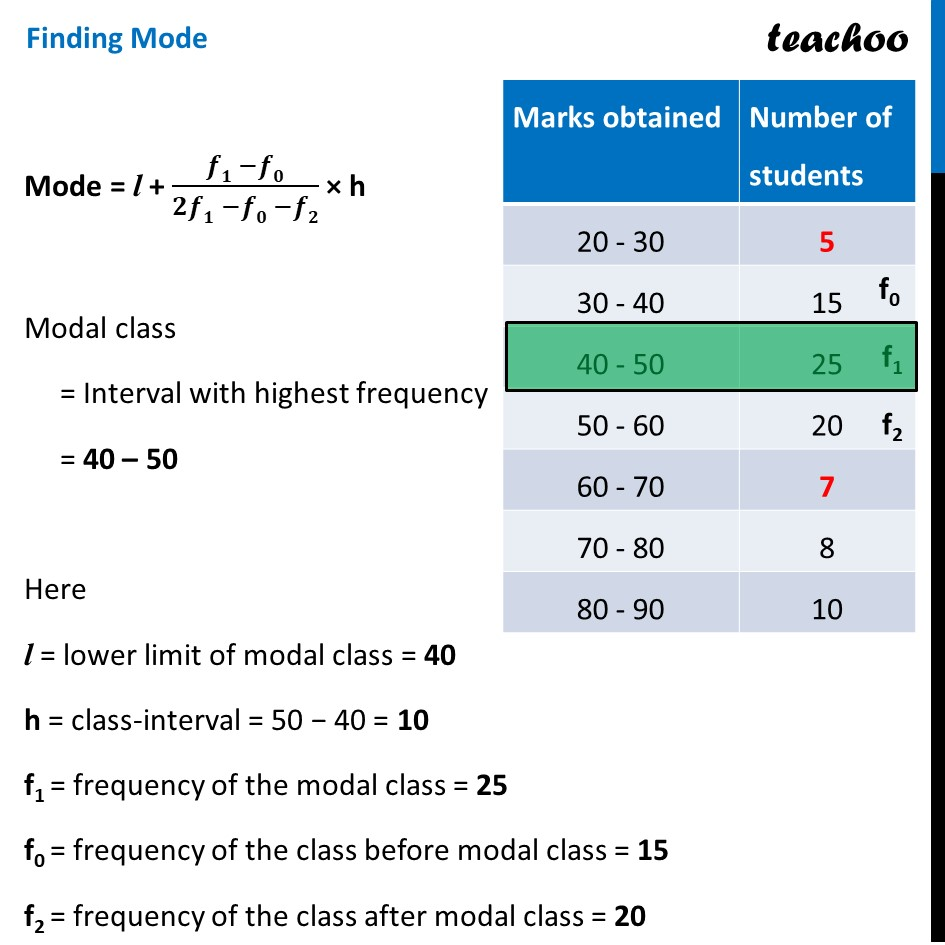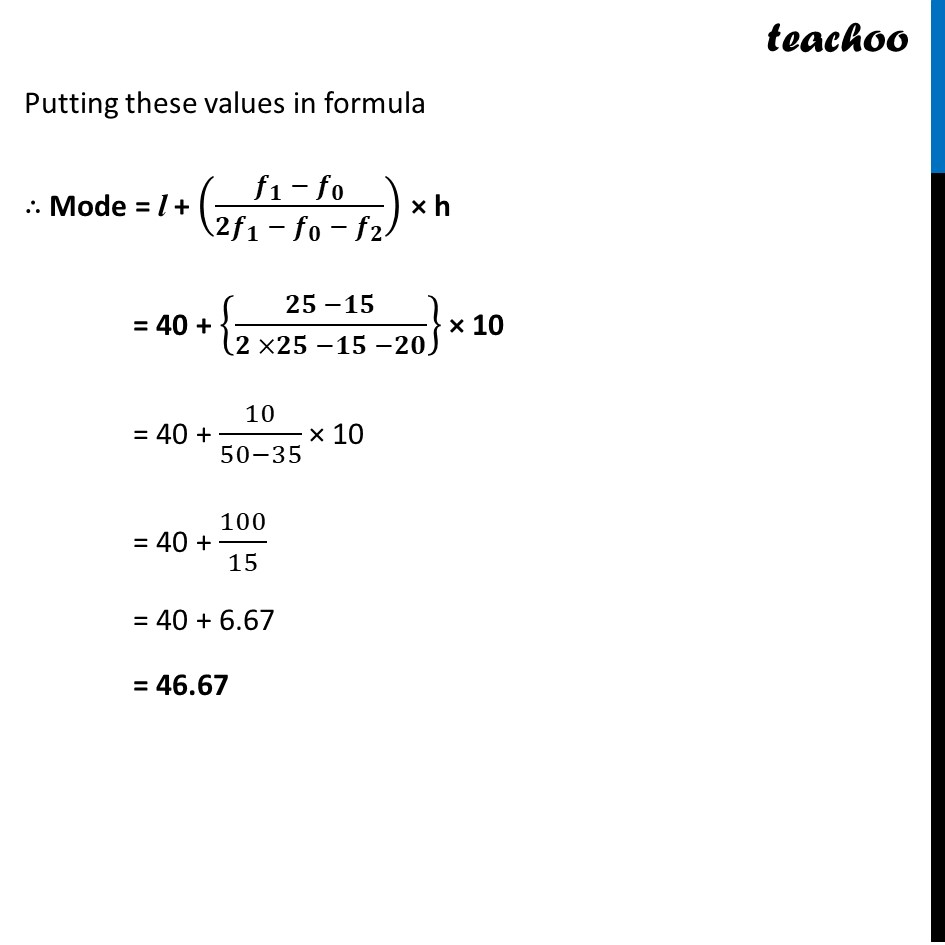Learn in your speed, with individual attention - Teachoo Maths 1-on-1 Class

### Transcript

Now, Median = l + (𝑵/𝟐 −𝒄𝒇)/𝒇 × h Since Median = 50 50 – 60 is Median Class Where N = ∑▒𝑓𝑖 l = h = cf = f = Putting values in formula Median = l + (𝑵/𝟐 −𝒄𝒇)/𝒇 × h 50 = 50 + (𝟒𝟓 − (𝒑 + 𝟒𝟎))/𝟐𝟎 × 10 50 = 50 + (5 −𝑝)/2 50 – 50 = (5 −𝑝)/2 0 = (5 −𝑝)/2 5−𝑝 = 0 p = 5 Also, ∑▒𝒇𝒊 = 78 + p + q Putting values 90 = 78 + 5 + q 90 = 83 + y 90 – 83 = q 7 = q q = 7 Hence p = 5, q = 7 Finding Mode Mode = l + (𝒇𝟏 −𝒇𝟎)/(𝟐𝒇𝟏 −𝒇𝟎 −𝒇𝟐) × h Modal class = Interval with highest frequency = 40 – 50 Here l = lower limit of modal class = 40 h = class-interval = 50 − 40 = 10 f1 = frequency of the modal class = 25 f0 = frequency of the class before modal class = 15 f2 = frequency of the class after modal class = 20 Putting these values in formula ∴ Mode = l + ((𝒇_𝟏 − 𝒇_𝟎)/(𝟐𝒇_𝟏 − 𝒇_𝟎 − 𝒇_𝟐 )) × h = 40 + {(𝟐𝟓 −𝟏𝟓)/(𝟐 ×𝟐𝟓 −𝟏𝟓 −𝟐𝟎)} × 10 = 40 + 10/(50−35) × 10 = 40 + 100/15 = 40 + 6.67 = 46.67